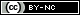## Articulo

•Citado por SciELO
•Accesos

•Similares en SciELO

## versión On-line ISSN 2521-9766versión impresa ISSN 1405-3195

#### Resumen

LOPEZ-CRUZ, Irineo L.; SALAZAR-MORENO, Raquel; ROJANO-AGUILAR, Abraham  y  RUIZ-GARCIA, Agustín. Global sensitivity analysis of a greenhouse lettuce (Lactuca sativa L.) crop model. Agrociencia [online]. 2012, vol.46, n.4, pp.383-397. ISSN 2521-9766.

Sensitivity analysis of a mathematical model is relevant, since it determines how the uncertainty of the model outputs can be assigned to its variables of input. So far local methods are applied based on the calculation of partial derivatives for models of greenhouse crops. However, the main drawback of the local sensitivity analysis is that it provides information only at the base point where the derivatives are calculated, without taking into account the rest of the interval of variation of input factors. To overcome these limitations, approaches of global sensitivity analysis are being developed such as scatter plots, standardized regression coefficients, methods based on the calculation of variances, the test of elementary effects and Monte Carlo filtering. In the present study, a global sensitivity analysis was performed based on variances to a greenhouse lettuce crop (Lactuca sativa L.) growth model. First, probability density functions were defined for all model parameters. Then, 5000 Monte Carlo simulations were developed by the Fourier amplitude sensitivity test (FAST) method to calculate the first-order sensitivity indices and those of total order. With Sobol's method 3000 Monte Carlo simulations were used to calculate both sensitivity indices. The Simlab program (version 3.2) was used for sensitivity analysis and Matlab to perform all simulations. Both the FAST and Sobol method allowed to determine that the most important parameters for total dry biomass of the model are the leaf conductance coefficient of CO2 (σ), photosynthetic efficiency coefficient (ε), the reference temperature (T*), the osmotic pressure of the vacuoles (πv) and the maintenance respiration coefficient (k).

Palabras llave : probability density function; sampling method; dynamic model; simulation; Lactuca sativa L..

· resumen en Español     · texto en Español     · Español (pdf )Todo el contenido de esta revista, excepto dónde está identificado, está bajo una Licencia Creative Commons